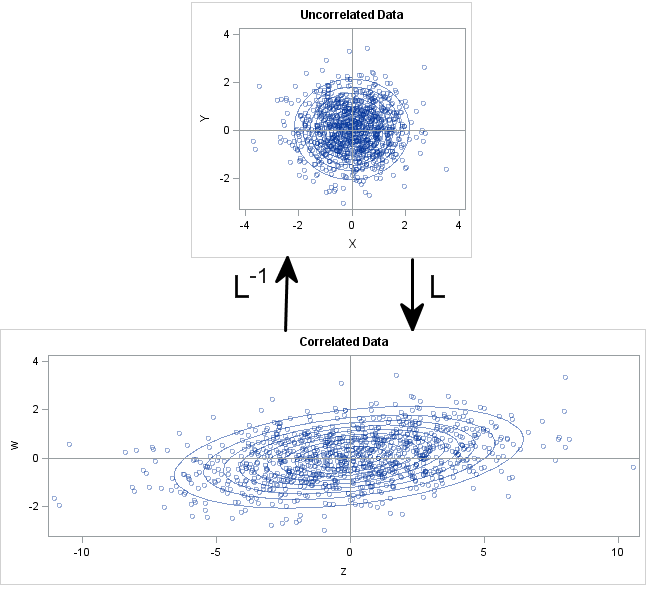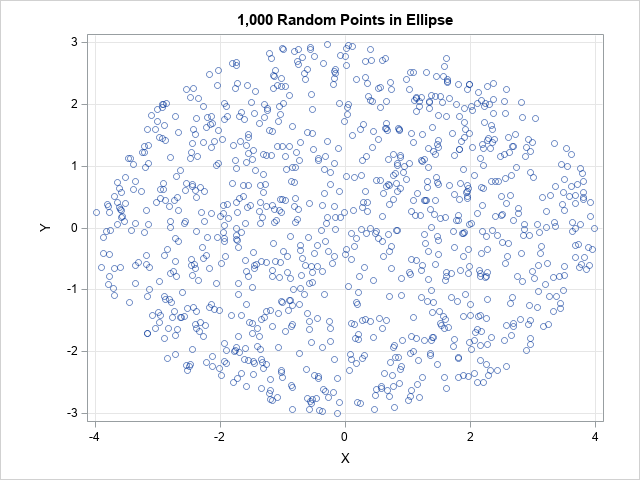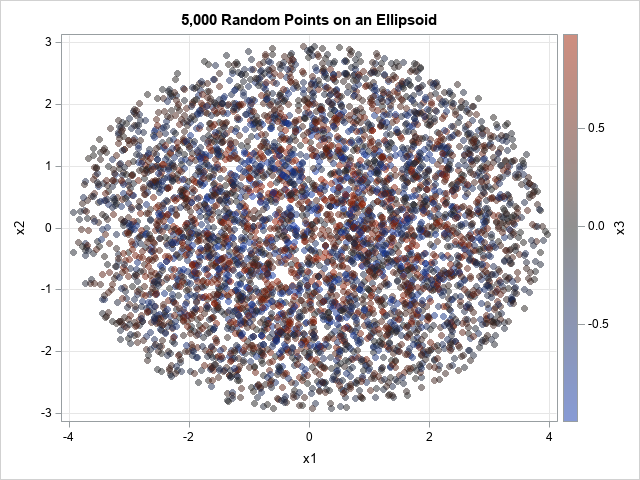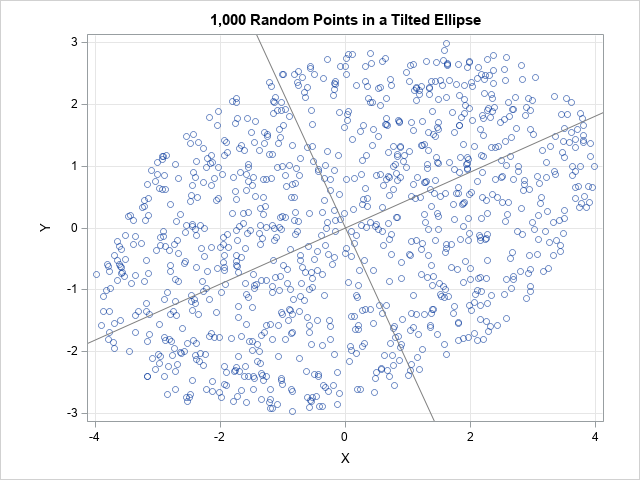I have previously written about how to efficiently generate points uniformly at random on the surface of a sphere. The method uses a mathematical fact from multivariate statistics: If X is drawn from the uncorrelated multivariate normal distribution, then S = X / ||X|| has the uniform distribution on the unit sphere. This is true in any dimension; see the previous article for pictures of a uniform sample on the circle in the plane and on a sphere in 3-D.

It is worth mentioning that this procedure can also be used to generate random points uniformly on any ellipse. This article shows the mathematical algorithm and implements it in SAS. Examples are shown in 2-D (an ellipse in the plane) and in 3-D (an ellipsoid). The same process works for hyperellipsoids in high-dimensional Euclidean space.

### From spheres to ellipsoidsIntuitively, you can move from spheres to ellipsoids by changing the covariance matrix that you use for the multivariate normal (MVN) distribution. When the covariance matrix is the identity matrix, the equi-probability contours of the density are spheres. However, for a general positive definite covariance matrix, the equi-probability contours of the density are (hyper)ellipsoids. A particularly simple choice for a covariance matrix is a diagonal matrix. For a diagonal matrix, the axes of the density ellipsoids are orthogonal and are aligned with the standard coordinate axes.

There is a simple geometric relationship between the equi-probability contours for an uncorrelated MVN distribution and for a correlated MVN distribution with covariance matrix Σ. Briefly, if you use the Cholesky factorization to decompose Σ = LLT, then the matrix L is a linear transformation that maps the spherical contours of the uncorrelated distribution to corresponding contours of the correlated distribution, as shown by the image to the right.

The geometry (and the computation) is simplest when you use a diagonal covariance matrix. If $\Sigma = \mbox{diag}(\sigma_1^2, \sigma_2^2, \ldots, \sigma_d^2)$ is a diagonal covariance matrix, then L = diag(σ1, σ2, ..., σd) for σi > 0. The linear transformation, L, maps the unit sphere at the origin to an ellipsoid with axes that are aligned with the standard coordinate axes and whose radii are σ1, σ2, ..., σd.

### The uniform distribution is preserved under a linear transformation

Of course, this problem requires more than mapping a sphere to an ellipsoid. We want to generate uniform random variates on the ellipsoid. Fortunately, you can prove that the uniform distribution is preserved under a linear transformation. More precisely, suppose that X is a continuous random variable with a uniform distribution on some subset S in d-dimensional Euclidean space. Let Y = μ + L*X be any affine transformation of X, where μ is a d-dimensional vector and L is an invertible d x d matrix. Then Y is uniformly distributed on the image of S, which is the set {y = μ + L*x | x ∈ S}.

This theorem tells you that you can generate random uniform variates on the unit sphere (as shown in the previous article) and then linearly transform those variates to obtain uniform variates on an ellipsoid. The transformed variates will be uniformly distributed on the image of the sphere, which is an ellipsoid.

### A SAS program to generate random uniform variates on an ellipse

You can carry out this process in any matrix-vector language, such as R, MATLAB, or SAS IML. The following SAS IML program defines a function that takes two arguments: the number of points (n) to generate, and a vector of d positive values, which represent the elements of a diagonal covariance matrix. It returns n points in d-dimensional space that are on the ellipse {y = diag(σ1, σ2, ..., σd)*x | x is on the unit sphere and σi > 0}.

/* Uniform random points on ellipsoid in d-dimensional space. The ellipsoid is the image of the unit sphere under the transformation x -> L*x where x is on the unit sphere and L = diag(sigma_1, sigma_2, ..., sigma_d), sigma_i > 0. */ proc iml; /* generate n random uniform points on the ellipse L*x, where x is on unit sphere and L = diag(s) */ start RandEllipse(n, s); d = ncol(s); mu = j(1, d, 0); X = randnormal(n, mu, I(d)); /* X ~ N(0, I) */ Z = X / sqrt( X[,##] ); /* Z is uniform on circle/sphere */ L = diag(s); /* Cholesky root of covariance matrix Sigma=diag(s##2) */ return Z * L; /* points are uniform on ellipse/ellipsoid */ finish;   call randseed(12345); /* 2-D example with n=200 points on ellipse in plane */ n = 200; sigmas = {4 3}; Y = RandEllipse(n, sigmas); title "200 Random Points on Ellipse"; call scatter(Y[,1], Y[,2]) grid={x y} procopt="aspect=0.75" option="transparency=0.5";A scatter plot of the output shows that the 200 points are distributed uniformly at random on the ellipse with one axis aligned with the vector (4,0) and the other axis aligned with the vector (0,3).

The same function supports generating points on higher-dimensional ellipsoids. For example, to get 1,000 random points on an ellipsoid in 3-D, you can use the following statements.

/* 3-D example with n=1,000 points on ellipsoid */ Y = RandEllipse(1000, {4 3 1}); /* axes aligned with (4,0,0), (0,3,0), and (0,0,1) */   /* write points to a data set and visualize */ create RandEllipsoid from Y[c={'x1' 'x2' 'x3'}]; append from Y; close; QUIT;   title "1,000 Random Points on an Ellipsoid"; proc sgplot data=RandEllipsoid; scatter x=x1 y=x2 / colorresponse=x3 transparency=0.5 markerattrs=(symbol=CircleFilled); xaxis grid; yaxis grid; run;The graph projects the points onto the (x,y) plane. The markers are color-coded according to the z value, which ranges in [-1,1]. Because the points are on an ellipsoid, points near (x,y)=(0,0) have z coordinates near ±1. Points whose values are close to z=0 are located near the ellipse that passes through (±4, 0) and (0, ±3).

### Ellipsoids not aligned with the coordinate axis

The same technique works even if you do not use a diagonal covariance matrix. Given any positive definite matrix, you can find the Cholesky decomposition Σ = LLT and use L to map random variate from the circle onto an ellipse. The following SAS IML function implements this general technique:

  proc iml; /* generate n random uniform points on the ellipse L*x, where x is on unit sphere and L satisfies Sigma = L*L */ start RandEllipseCov(n, Sigma); d = ncol(Sigma); mu = j(1, d, 0); X = randnormal(n, mu, I(d)); /* X ~ N(0, I) */ Z = X / sqrt( X[,##] ); /* Z is uniform on circle/sphere */ U = root(Sigma); /* Cholesky decomp Sigma=U*U, U upper triangular */ L = U; /* Sigma=L*L', L lower triangular */ return Z * L; /* points are uniform on ellipse/ellipsoid */ finish;   call randseed(12345); /* 2-D example with n=200 points on ellipse in plane */ n = 200; Sigma = {16 4, 4 9}; Y = RandEllipseCov(n, Sigma);   title "200 Random Points on Tilted Ellipse"; /* slopes of reference lines are the directions of the eigenvectors of Sigma */ call scatter(Y[,1], Y[,2]) grid={x y} procopt="aspect=0.75 noautolegend" option="transparency=0.5" other="lineparm x=0 y=0 slope=0.4538/ lineattrs=(color=gray); lineparm x=0 y=0 slope=-2.2038/lineattrs=(color=gray);";`The graph overlays two reference lines, which indicate the axes for the ellipse. They are computed by using the eigenvectors of the Σ matrix. The computation is not shown here, but you can read the details in a previous article about ellipses and covariance matrices.

### Summary

This article shows how to generate points uniformly at random on an ellipse, on an ellipsoid, or on any hyperellipsoid in higher dimensions. It first generates points uniformly at random on the unit sphere, then uses a linear transformation to map the points onto an ellipsoid. If you want an ellipse whose axes are aligned with the coordinate axes, the linear transformation is given by a simple diagonal matrix with positive values on the diagonal. You can also generate points on ellipsoids whose axes are not aligned with the coordinate axes. If Σ is any positive definite matrix, you can decompose Σ = LLT. If you generate points on the unit sphere, you can use L to map them onto the ellipse whose axes are aligned with the eigendirections of Σ.

ShareDistinguished Researcher in Computational Statistics

Rick Wicklin, PhD, is a distinguished researcher in computational statistics at SAS and is a principal developer of SAS/IML software. His areas of expertise include computational statistics, simulation, statistical graphics, and modern methods in statistical data analysis. Rick is author of the books Statistical Programming with SAS/IML Software and Simulating Data with SAS.

1.Thanks, Rick, for this interesting sequel to your previous article.

I'm not sure I understand your reasoning in section "The uniform distribution is preserved under a linear transformation." The statement in the linked reference (about the uniform distribution being preserved) does not apply to the (d-1)-dimensional sphere S because its d-dimensional volume is zero. Indeed, in the first 2-D example the probability densities near (-4, 0) and (4, 0) are greater than those near (0, 3) and (0, -3), resulting in different expected numbers of random points per unit arc length. Also, in my histograms the marginal distributions of the x- and y-coordinates don't look uniform, but rather U-shaped. (As I don't have a SAS/IML license, I used a data step to reproduce the ellipse dataset. Thanks to the common "MTHybrid" random-number generator I obtained the same 200 points.)

•In any random sample from a uniform distribution, there will be gaps and clusters. If you change the random number seed, the gaps and clusters will move to other locations. If you run my first example several times with different seeds, you will see that there is no systematic bias in the distribution of the points.

As to your comment about the marginal histogram, yes, the marginal histogram should be U-shaped. Uniformity on the ellipse does not imply uniformity when the points are projected onto the X or Y axis. In fact, you can prove that if (x,y) is uniformly distributed on the unit circle, that the marginal distribution has the density f(x) = 1/ (pi*sqrt(1-x^2)), which is a U-shaped density on the interval (-1,1).

•Thanks for your response. I mentioned the (non-uniform) marginal distributions because you wrote: "You can use histograms to demonstrate that each coordinate has a uniform marginal distribution." Maybe I have misunderstood this sentence.

My first issue is not about the random gaps and clusters of the empirical distribution, but really about the theoretical distribution. It helped me to envision a uniform distribution on a square (rather than a circle), e.g., S={(x, y) in [-1, 1]²: |x|=1 or |y|=1}. Now, if the linear transformation Y = diag(1, 0.5)*X is applied to this square, isn't the probability density on the vertical sides (length=1) of the resulting rectangle twice as large as on the horizontal sides (length=2)? A similar effect should occur when a circle is transformed to an ellipse: The transformation with diag(1, 0.5) -- or even diag(1, 0.001) -- should lead to an increased probability density near the left and right vertices of the resulting ellipse (in comparison to the co-vertices), hence a non-uniform distribution if the distribution on the circle was uniform.

•I don't know why I wrote that sentence. It is not correct. Thanks for pointing out my mistake.

Regarding the square and the linear transformation, yes, what you describe is correct. But the term "uniform density" depends on the domain, not just the density function.
For the interval [0,1], the function f(x)=1 is the uniform density function. If I stretch the domain by a factor of 2, then the function g(y)=1/2 is the uniform density on [0,2]. You can read about how to convert one density into another under a linear transformation. For this example, with L=2 being the linear transformation, we have g(y) = (1/L) f(y/L).

•I agree that the term "uniform density" depends on the domain. But isn't the characteristic feature of a uniform distribution that the density is constant on its domain (up to null sets)? The distribution in my example is not uniform on the rectangle (where the density takes two different values), so the original uniform distribution on the square was not preserved under the linear transformation. (Which does not contradict the theorem you referred to because it does not apply to null sets such as squares or circles as subsets of the plane.)

The original probability distributions on your circles and spheres are uniform. My point is that the distributions on the ellipses and ellipsoids you obtained by affine transformations are not uniform in the above sense. Their densities vary as you move around those curves/surfaces. As a consequence, when sampling from these distributions, the expected number of random points per unit arc length (surface area or (d-1)-dimensional volume in the general case) varies in the same way. On average, the number of random points in a small neighborhood of one of the vertices of the ellipse (e.g., the image of the circle under the diag(1, 0.5) transformation) will be greater than the corresponding number in a neighborhood of the same size (i.e., arc length) of a point anywhere else on the ellipse.

In what sense are these distributions uniform?